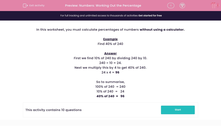# Numbers: Working Out the Percentage

In this worksheet, students work out a percentage of a number without using a calculator.Key stage:  KS 2

Curriculum topic:   Maths and Numerical Reasoning

Curriculum subtopic:   Percentages

Difficulty level:#### Worksheet Overview

In this worksheet, you must calculate percentages of numbers without using a calculator.

Example

Find 40% of 240

First we find 10% of 240 by dividing 240 by 10.

240 ÷ 10 = 24.

Next we multiply this by 4 to get 40% of 240.

24 x 4 = 96

So to summarise,

100% of 240 = 240

10% of 240 =   24

40% of 240 =   96

### What is EdPlace?

We're your National Curriculum aligned online education content provider helping each child succeed in English, maths and science from year 1 to GCSE. With an EdPlace account you’ll be able to track and measure progress, helping each child achieve their best. We build confidence and attainment by personalising each child’s learning at a level that suits them.

Get started••••